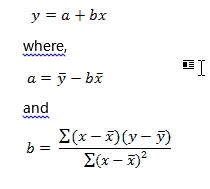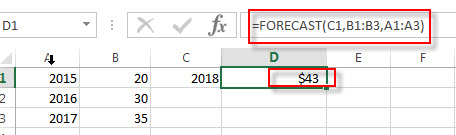# Excel FORECAST Function

This post will guide you how to use the FORECAST function with syntax and examples in Microsoft excel.

### Description

The Excel FORECAST function used to calculate or predict a future value by using existing values. You can use the FORECAST function to get the predicated y-value for a given x-value. So the known values are existing x-values and y-values, and then get the new predicted by using linear regression.

The FORECAST function has been replaced by the FORECAST.LINEAR function in Excel 2016.

For example, you can use this function to predict future sales trends.

The FORECAST function is a build-in function in Microsoft Excel and it is categorized as a Statistical Function.

The FORECAST function is available in Excel 2016, Excel 2013, Excel 2010, Excel 2007, Excel 2003, Excel XP, Excel 2000, Excel 2011 for Mac.

### Syntax

The syntax of the FORECAST function is as below:

`= FORECAST (x, known_y's, known_x's)`

Where the FORECAST function arguments are:

• X -This is a required argument.  A numeric x-value that used to predict the new y-value.
• known_y’s -This is a required argument.  The known y-values or range of data used to predict the new y-value.
• known_x’s -This is a required argument.  The known x-values or range of data used to predict the new y-value.

Note:

• If X argument is non-numeric, the FORECAST Function will return the #VALUE! Error value.
• If Known_y’s and known_x’s arguments are empty or contain a different array lengths, the FORECAST function will return #N/A Error. It means that the length of the known_y’s array must be same as the known_y’s length.
• If the variance of the known_x’s is equal to 0, the FORECAST function will return the #DIV/0! Error value.
• The FORECAST function try to calculate a new y-value using the following equation:### Excel FORECAST Function Examples

The below examples will show you how to use Excel FORECAST Function to calculate a prediction of a future value based on existing values.

1# to predict a future value based on known x-values A1:A3 and known y-values B1:B3, using the following formula:

`=FORECAST(C1,B1:B3,A1:A3)`### Related Functions

• Excel GROWTH Function
The Excel GROWTH function calculates the predicted exponential growth based on existing data. The syntax of the GROWTH function is as below:= GROWTH (known_y’s, [known_x’s], [new_x’s], [const])…